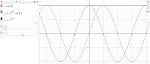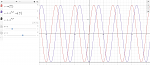# Getting a converging solution to a second-order differential equation

#### enea19

##### New member
I have been struggling with a problem for a long time. I need to solve the second order partial differential equation

$$\displaystyle \frac{1}{G_{zx}}\frac{\partial ^2\phi (x,y)}{\partial^2 y}+\frac{1}{G_{zy}}\frac{\partial ^2\phi (x,y)}{\partial^2 x}=-2 \theta$$

where $$\displaystyle G_{zx}$$, $$\displaystyle G_{zy}$$, $$\displaystyle \theta$$, $$\displaystyle a$$, and $$\displaystyle b$$ are constants and with BCs $$\displaystyle \phi (0,y)=\phi (a,y)=0$$ and $$\displaystyle \phi (x,-b)=\phi (x,b)=0$$. The solution that I have sets $$\displaystyle \phi (x,y)=\sum _{k=1,3,5\text{...}}^{\infty } Y(y) \sin \left(\frac{\pi k}{a}x\right)$$ and expands $$\displaystyle -2 \theta$$ in a Fourier sine series in the interval between 0 and a so we end up with a second order differential equation,

$$\displaystyle \frac{Y''(y)}{G_{zx}}-\frac{\pi ^2 k^2 Y(y)}{a^2 G_{zy}}=-\frac{8 \theta }{\pi k}$$

I tried several times to solve it by hand but end up making mistakes. Therefore I resorted to Mathematica and it gives me the following solution,

$$\displaystyle \phi_{mine}(x,y)=-\sum_{k=1,3,5,...}^{\infty}\frac{8 a^2 G_{zy} \theta \left(\text{sech}\left(\frac{\pi b k}{2 a} \frac{\sqrt{G_{zx}}}{\sqrt{G_{zy}}} \right) \cosh \left(\frac{\pi k y}{a} \frac{\sqrt{G_{zx}}}{\sqrt{G_{zy}}}\right)-1\right)}{\pi ^3 k^3}\sin \left(\frac{\pi k x}{a}\right)$$

This is almost exactly what is written in the solution that I have, which is

$$\displaystyle \phi_{sol}(x,y)=\frac{8}{\pi^3} G_{zy} a^2 \sum_{k=1,3,5,...}^{\infty}\frac{(-1)^{(k-1)/2}}{k^3}\left( 1-\frac{\cosh \left(\frac{\pi k \mu }{a}y\right)}{\cosh \left(\frac{b \pi k \mu}{2 a}\right)} \right)\cos \left(\frac{\pi k}{a}x\right)$$

where $$\displaystyle \mu=\sqrt{\frac{G_{zx}}{G_{zy}}}$$. $$\displaystyle \phi_{sol}(x,y)$$ is missing \theta but I suspect that it might be a typo.

I know that unfortunately $$\displaystyle \phi_{mine}(x,y)$$ is wrong because the next step in the process involves working out a constant $$\displaystyle \beta$$ where

$$\displaystyle \beta=\frac{2 \int_{-b/2}^{b/2} \left(\int_0^a \phi (x,y) \, dx\right) \, dy}{G_{zx} a b^3}$$

When I work out $$\displaystyle \beta$$ I get that $$\displaystyle \phi_{sol}(x,y)$$ converges to a value while $$\displaystyle \phi_{mine}(x,y)$$ goes to infinity. Therefore I'm doing something wrong but I'm not sure what. I've been trying to figure out the difference between my answer and the solution and all I can find is that somehow

$$\displaystyle \sin \left(\frac{\pi k x}{a}\right)=\cos\left(\frac{\pi k x}{a}\right) (-1)^{(k-1)/2}$$

for $$\displaystyle k=1,3,5,...$$ Is it possible to change $$\displaystyle \sin \left(\frac{\pi k x}{a}\right)$$ into $$\displaystyle \cos\left(\frac{\pi k x}{a}\right) (-1)^{(k-1)/2}$$ when only odd values of $$\displaystyle k$$ are used? I've never seen this and when I plot them they give different curves so they don't seem to be equivalent.